# Chessboard paradox facts for kids

Kids Encyclopedia Facts

The chessboard paradox or paradox of Loyd and Schlömilch is a falsidical paradox based on an optical illusion. A chessboard or a square with a side length of 8 units is cut into four pieces. Those four pieces are used to form a rectangle with side lengths of 13 and 5 units. Hence the combined area of all four pieces is 64 area units in the square but 65 area units in the rectangle, this seeming contradiction is due an optical illusion as the four pieces don't fit exactly in the rectangle, but leave a small barely visible gap around the rectangle's diagonal. The paradox is sometimes attributed to the American puzzle inventor Sam Loyd (1841–1911) and the German mathematician Oskar Schlömilch (1832–1901)

## Generalisation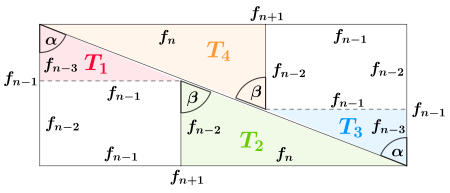: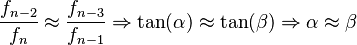$\frac{f_{n-2}}{f_n} \approx \frac{f_{n-3}}{f_{n-1}} \Rightarrow \tan(\alpha) \approx \tan(\beta) \Rightarrow \alpha \approx \beta$,
the triangles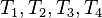$T_1, T_2, T_3, T_4$ are nearly similar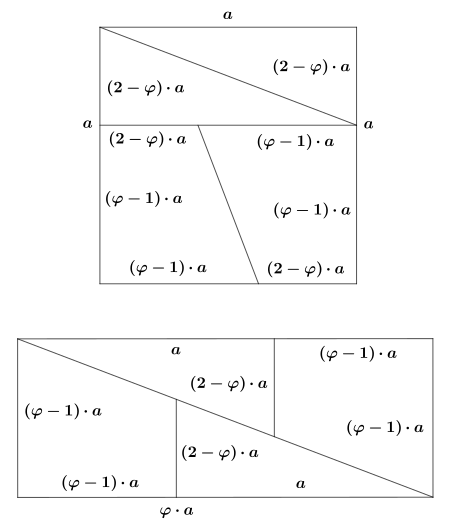dissection without an area mismatch$\varphi=\frac{1+\sqrt{5}}{2}$

The line segments occurring in the drawing of the last chapters are of length 2, 3, 5, 8 and 13. These are all sequential Fibonacci numbers, suggesting a generalisation of the dissection scheme based on Fibonacci numbers. The properties of the Fibonacc numbers also provide some deeper insight, why the optical illusion works so well. A square whose side length is the Fibonacci number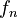$f_n$ can be dissected using line segments of lengths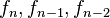$f_n, f_{n-1}, f_{n-2}$ in the same way the chessboard was dissected using line segments of lengths 8, 5, 3 (see graphic).

Cassini's identity states: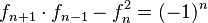$f_{n+1} \cdot f_{n-1} - f_n^2=(-1)^n$

From this it is immediately clear that the difference in area between square and rectangle must always be 1 area unit, in particular for the original chessboard paradox one has: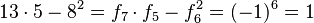$13 \cdot 5 -8^2= f_7 \cdot f_5 - f_6^2=(-1)^6=1$

Note than for an uneven index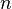$n$ the area of the square is not smaller by one area unit but larger. In this case the four pieces don't create a small gap when assembled into the rectangle, but they overlap slightly instead. Since the difference in area is always 1 area unit the optical illusion can be improved using larger Fibonacci numbers allowing gap's percentage of the rectangle area to become arbitrarily small and hence for practical purposes invisible.

Since the ratio of neighbouring Fibonacci numbers converges rather quickly against the golden ratio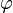$\varphi$, the following ratios converge quickly as well: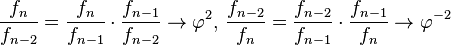$\frac{f_n}{f_{n-2}}= \frac{f_n}{f_{n-1}} \cdot \frac{f_{n-1}}{f_{n-2}}\rightarrow \varphi^2,\, \frac{f_{n-2}}{f_n}= \frac{f_{n-2}}{f_{n-1}} \cdot \frac{f_{n-1}}{f_n}\rightarrow \varphi^{-2}$

For the four cut-outs of the square to fit together exactly to form a rectangle the small parallelogram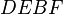$DEBF$ needs to collapse into a line segment being the diagonal of the parallelogram. In this case the following holds for angles in the rectangle due to being corresponding angles of parallels: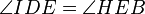$\angle IDE =\angle HEB$,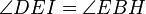$\angle DEI =\angle EBH$,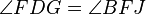$\angle FDG =\angle BFJ$,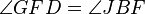$\angle GFD =\angle JBF$

As a consequence the following right triangles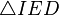$\triangle IED$,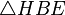$\triangle HBE$ ,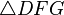$\triangle DFG$ and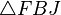$\triangle FBJ$ must be similar and the ratio of their legs must be the same.

Due to the quick convergence stated above the according ratios of Fibonacci numbers in the assembled rectangle are almost the same: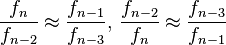$\frac{f_n}{f_{n-2}} \approx \frac{f_{n-1}}{f_{n-3}},\, \frac{f_{n-2}}{f_n} \approx \frac{f_{n-3}}{f_{n-1}}$

Hence they almost fit together exactly, this creates the optical illusion.

One can also look at the angles of the parallelogram as in the original chessboard analysis. For those angles the following formulas can derived: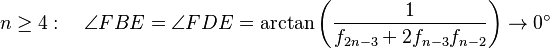$n\geq 4:\quad\angle FBE = \angle FDE=\arctan\left( \frac{1}{f_{2n-3}+2f_{n-3}f_{n-2}}\right)\rightarrow 0^\circ$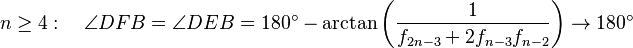$n\geq 4:\quad\angle DFB = \angle DEB=180^\circ - \arctan\left( \frac{1}{f_{2n-3}+2f_{n-3}f_{n-2}}\right)\rightarrow 180^\circ$

Hence the angles converge quickly towards the values needed for an exact fit.

It is however possible to use the dissection scheme without a creating an area mismatch, that is the four cut-outs will assemble exactly into an rectangle of the same area as the square. Instead of using Fibonacci numbers one bases the dissection directly on the golden ratio itself (see drawing). For a square of side length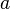$a$ this yields for the area of the rectangle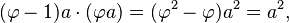$(\varphi-1)a \cdot (\varphi a)=(\varphi^2-\varphi)a^2=a^2,$

since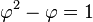$\varphi^2-\varphi=1$ is a property of the golden ratio.Chessboard paradox Facts for Kids. Kiddle Encyclopedia.